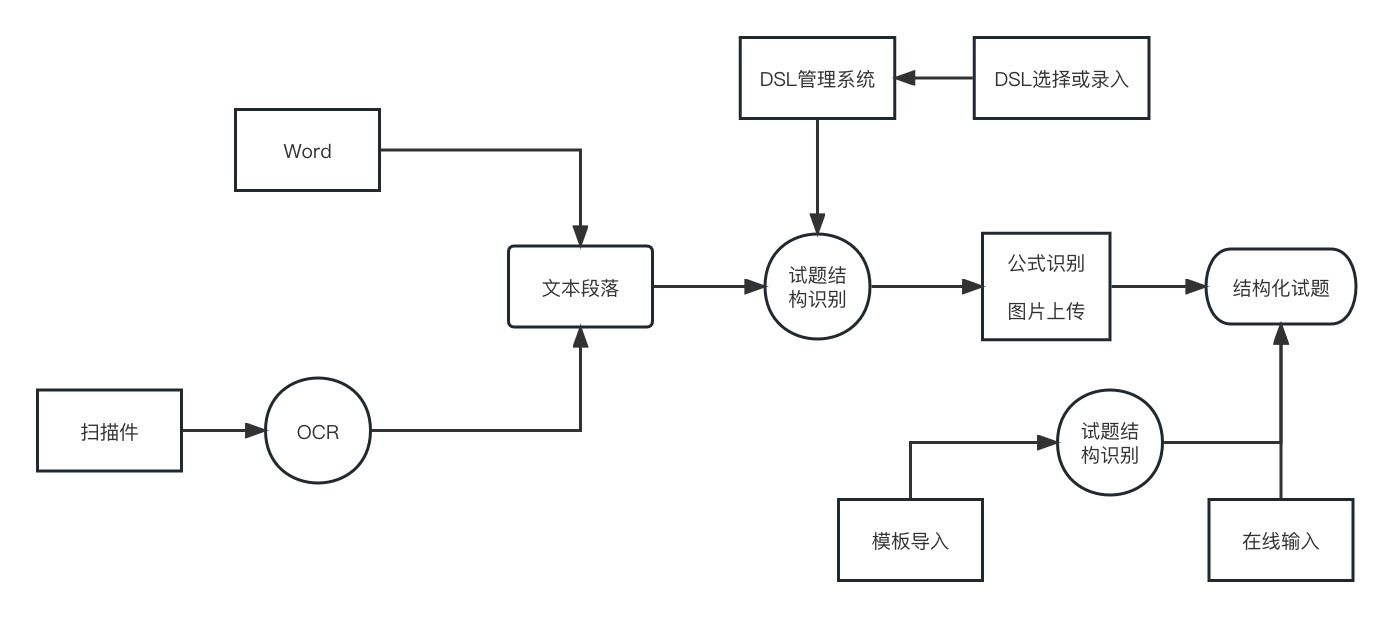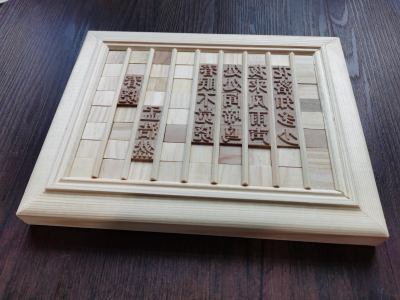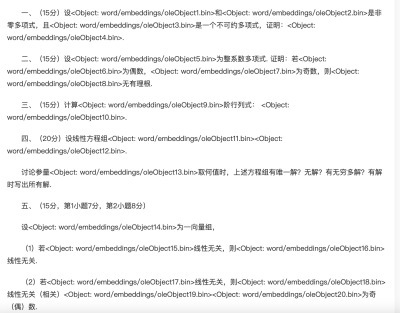# 题库系统设计

• 录入

• 标准化录入
• 模板录入(word,excel等)录入
• 在线格式化录入
• 非标准化录入
• 第三方word录入
• 题目格式解析
• 公式识别
• 图片处理
• 扫描数据录入
• OCR识别
• 试题结构解析
• 存储

• 标准化格式处理
• 标签系统
• 检索系统
• 组卷

• 输出Word
• 输出pdf
• 输出Tex

## 试题录入## 存储MathML全称为数学标记语言（Mathematical Markup Language），是一种基于XML的标准，用来在互联网上书写数学符号和公式的置标语言。MathML可以直接从HTML5使用MathML标签。 当您希望在网页中显示更多简单的数学符号时，它非常有用，并且由于其简单性和与HTML的相似性 ，因此非常易于使用。 比如勾股定理的mathml表示如下：

<?xml version="1.0" encoding="iso-8859-1"?>
<!DOCTYPE math PUBLIC "-//W3C//DTD MathML 2.0//EN""http://www.w3.org/TR/MathML2/dtd/mathml2.dtd">
<math xmlns="http://www.w3.org/1998/Math/MathML">
<msup>
<mi>a</mi>
<mn>2</mn>
</msup>
<mo>+</mo>
<msup>
<mi>b</mi>
<mn>2</mn>
</msup>
<mo>=</mo>
<msup>
<mi>c</mi>
<mn>2</mn>
</msup>
</math>


office用的是Office Open Xml, 它不是标准的xml，所以实现起来有些差异。一个n的额平分的OMML如下：

<m:oMathPara><!-- mathematical block container used as a paragraph -->
<m:oMath><!-- mathematical inline formula -->
<m:f><!-- a fraction -->
<m:num><m:r><m:t>π</m:t></m:r></m:num>
<m:den><m:r><m:t>2</m:t></m:r></m:den>
</m:f>
</m:oMath>
</m:oMathPara>

LaTeX

• 将数学公式写在 $ $ 之间，代表的是插入行内数学公式（通常称为行内模式）。
• 将数学公式写在 $$ $$ 之间，会使公式独立成一行并强制居中（通常称为独立模式）。
• 字体颜色：{\color{色调}表达式}
• 背景颜色：{\color{文字色调}\colorbox{背景色调}{表达式(可以打中文)}}
• latex跟word一样插入的图片是本地图片，如果要引入网络图片，需要\write18命令，如\write18{wget http://www.abc.com/path/to/image.png}